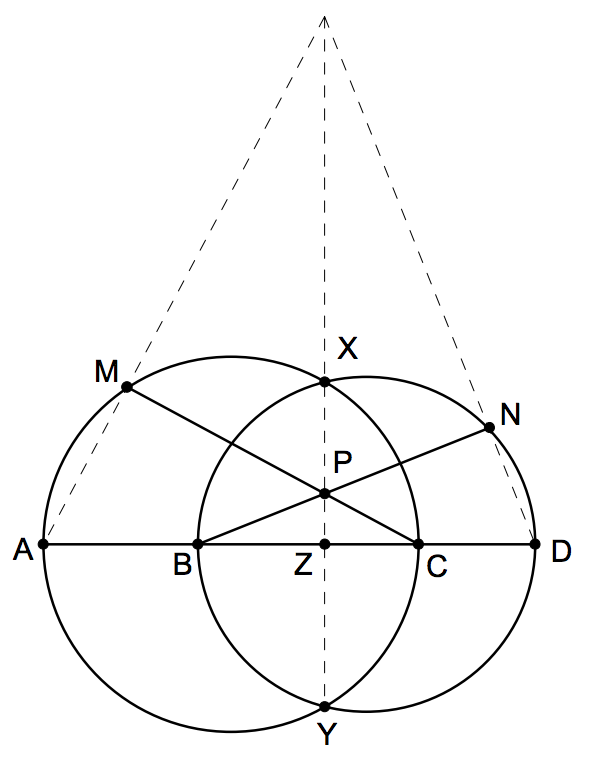#### Triangle IMO

###### back to index

Given an acute $\triangle{ABC}$ with side length $BC > CA$.

Let $A$, $B$, $C$ and $D$ be four distinct points on a line, in that order. The circles with diameters $AC$ and $BD$ intersect at the points $X$ and $Y$. The line $XY$ meets $BC$ at the point $Z$. Let $P$ be a point on the line $XY$ different from $Z$. The line $CP$ intersects the circle with diameter $AC$ at the points $C$ and $M$, and the line $BP$ intersects the circle with diameter $BD$ at the points $B$ and $N$. Prove that the lines $AM$, $DN$ and $XY$ are concurrent(Weitzenbock's Inequality) Let $a, b, c$, and $S$ be a triangle's three sides' lengths and its area, respectively. Show that $$a^2 + b^2 + c^2 \ge 4\sqrt{3}\cdot S$$

The diagonals $AC$ and $CD$ of the regular hexagon $ABCDEF$ are divided by inner points $M$ and $N$ such that $AM:AC = CN:CE=r$. Determine $r$ if $B, M,$ and $N$ are collinear.

Prove that there is one and only one triangle whose side lengths are consecutive integers, and one of whose angles is twice as large as another.